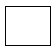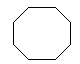Email us to get an instant 20% discount on highly effective K-12 Math & English kwizNET Programs!

#### Online Quiz (WorksheetABCD)

Questions Per Quiz = 2 4 6 8 10

### MEAP Preparation - Grade 4 Mathematics3.15 Regular Polygons

 A polygon in which all the sides are equal and all the angles are equal is called a regular polygon. Directions: Answer the following. Also draw at least five examples of regular polygon.
 Q 1: The figure shows regular triangle which is also calledisosceles triangleequilateral triangleright angle triangle Q 2: A regular quadrilateral is also called asquarerectangleparallelogram Q 3: The figure shown is a regularoctagonhexagonpentagon Q 4: The figure shown is a regularsquarepentagonoctagon Q 5: The figure shown is a regularhexagonoctagonpentagon Q 6: A regular polygon hassides of equal lengthangles of equal measuresides of equal length and angles of equal measure Question 7: This question is available to subscribers only! Question 8: This question is available to subscribers only!